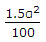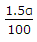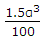# Civil Engineering - Surveying

Exercise : Surveying - Section 7
46.
If deflection angles are measured in a closed traverse, the difference between the sum of the right-hand and that of the left hand angles should be equal to
90°
180°
360°
Explanation:
No answer description is available. Let's discuss.

47.
Locating the position of a plane table station with reference to three known points, is known as
intersection method
resection method
three point problem.
Explanation:
No answer description is available. Let's discuss.

48.
If D is the degree of the curve of radius R, the exact length of its specified chord, is
radius of the curve x sine of half the degree
diameter of the curve x sine of half the degree
diameter of the curve x cosine of half the degree
diameter of the curve x tangent of half the degree.
Explanation:
No answer description is available. Let's discuss.

49.
Correction per chain length of 100 links along a slope of α° is1.5α3.